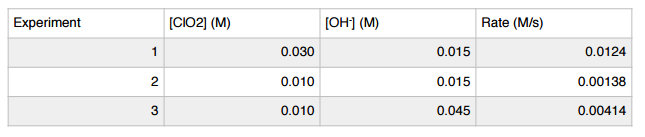# Problem: Determine the rate law for the reaction 2ClO2 (aq) + 2 OH - (aq) → ClO2- + H2O (l) given the following data.a. Rate = k[ClO2][OH-]b. Rate = k[ClO2]2[OH-]c. Rate = k[ClO2]2[OH-]2d. Rate = k[ClO2][OH-]2e. Rate = k[OH-]

###### FREE Expert Solution
86% (404 ratings)
###### Problem Details

Determine the rate law for the reaction 2ClO2 (aq) + 2 OH - (aq) → ClO2- + H2O (l) given the following data.

a. Rate = k[ClO2][OH-]

b. Rate = k[ClO2]2[OH-]

c. Rate = k[ClO2]2[OH-]2

d. Rate = k[ClO2][OH-]2

e. Rate = k[OH-]What scientific concept do you need to know in order to solve this problem?

Our tutors have indicated that to solve this problem you will need to apply the Rate Law concept. You can view video lessons to learn Rate Law. Or if you need more Rate Law practice, you can also practice Rate Law practice problems.

What is the difficulty of this problem?

Our tutors rated the difficulty ofDetermine the rate law for the reaction 2ClO2 (aq) + 2 OH - ...as medium difficulty.

How long does this problem take to solve?

Our expert Chemistry tutor, Sabrina took 7 minutes and 46 seconds to solve this problem. You can follow their steps in the video explanation above.

What professor is this problem relevant for?

Based on our data, we think this problem is relevant for Professor Patterson's class at TXSTATE.